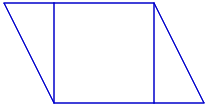### Home > CC2 > Chapter 9 > Lesson 9.1.3 > Problem9-46

9-46.

Draw a parallelogram with a base of $3$ cm and a height of $7$ cm and show how to calculate its area.

An example of a parallelogram is given below.
It is divided into triangles and a square.
You can use this strategy to find the area of a parallelogram.